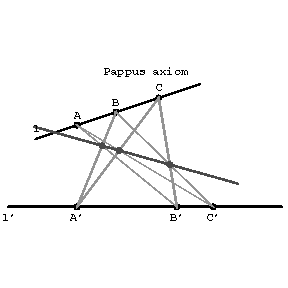# Pappus axiom

If \$l\$ and \$l'\$ are two distinct straight lines and \$A,B,C\$ and \$A',B',C'\$ are distinct points on \$l\$ and \$l'\$, respectively, and if none of these is the point of intersection of \$l\$ and \$l'\$, then the points of intersection of \$AB'\$ and \$A'B\$, \$BC'\$ and \$B'C\$, \$AC'\$ and \$A'C\$ are collinear.Figure: p071140a

The truth of Pappus' axiom is equivalent to the commutativity of the skew-field of the corresponding projective geometry. The Desargues assumption is a consequence of Pappus' axiom (Hessenberg's theorem), and at the same time Pappus' axiom is a degenerate case of the Pascal theorem. The axiom was proposed by Pappus (3rd century).